# 【扑克反水】德州扑克静态弃牌赢率&动态弃牌赢率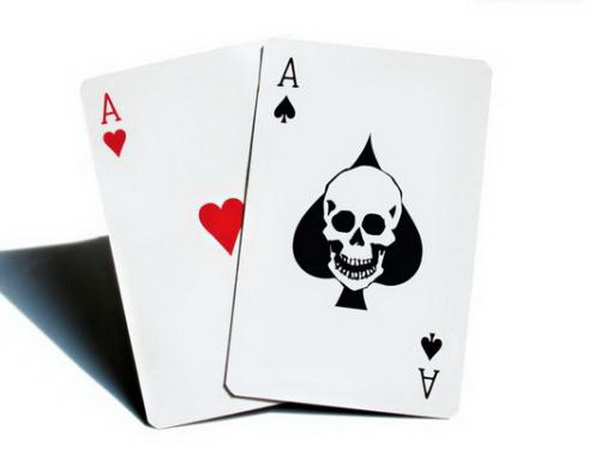100（初始底池金额）+ 100（你的全压金额）= 200

100（你的全压金额）/ 200（底池总额）= 盈亏平衡需要的弃牌率%

100 / 200 = 0.5 = 50%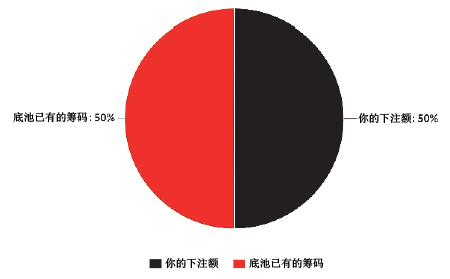100（初始底池金额）+ 50（你的全压金额）= 150

100（你的全压金额）/ 150（底池总额）= 盈亏平衡需要的弃牌率%

50 / 150 = 0.33 = 33%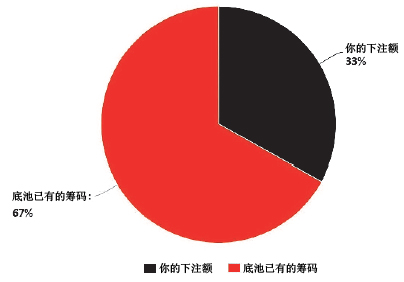100（初始底池金额）+ 200（你的全压金额）= 300

200（你的全压金额）/ 300（底池总额）= 盈亏平衡需要的弃牌率%

200 / 300 = 0.67 = 67%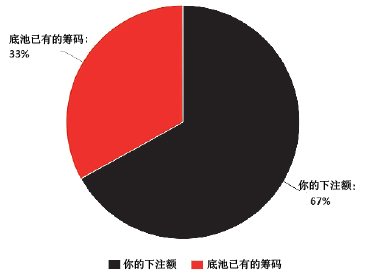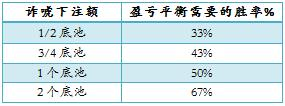EV = XP + (1 – X)(WH – LV)

X = 弃牌频率 = 40% = 0.2
P = 底池大小 = 100
W = 净利润 = 200
H = 我们的胜率 = 20% = 0.2
L = 亏损的金额 = 100
V = 对手的胜率 = 80% = 0.8
EV = XP + (1 – X)(WH – LV)
= 0.4 x 100 + (1 – 0.4)(200 x 0.2 – 100 x 0.8)
= 40 + 0.6 x (40 – 80)
= 40 + 0.6 x (-40)
= 40 + (-24)
= 16

0 = XP + (1 – X)(WH – LV)
0 = 100X + (1 – X)(200 x 0.2 – 100 x 0.8)
0 = 100X + (1 – X)(40 – 80)
0 = 100X + (1 – X)(-40)
0 = 100X – 40 + 40X
40 = 100X + 40X
40 = 140X
140X = 40
X = 40/140
X = 4/14

X = 0.29或29%

http://www.bogoupoker.com